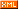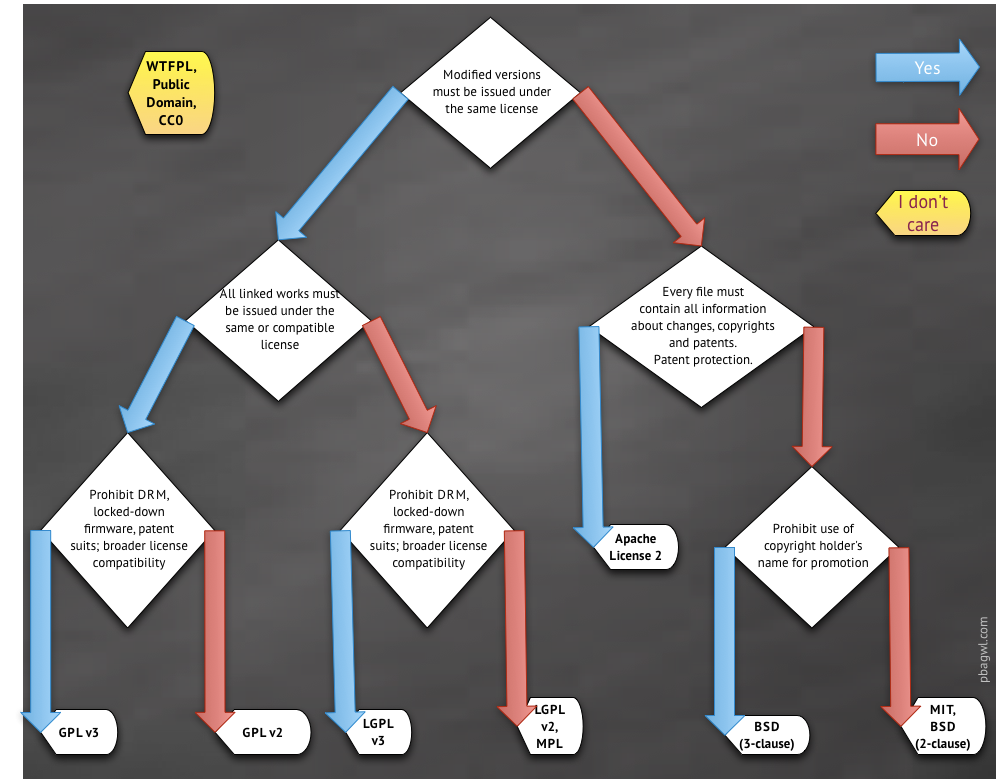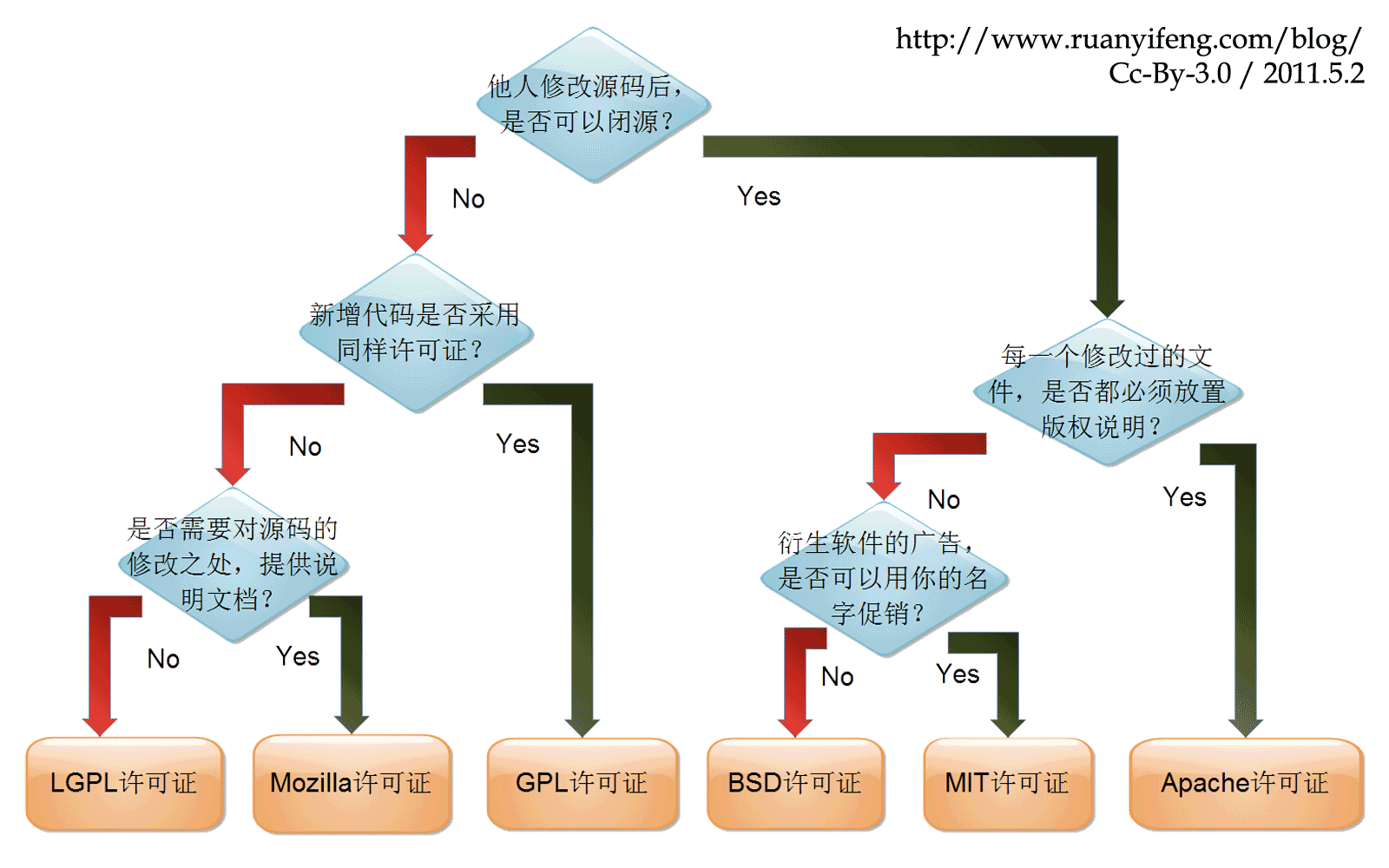# 数据库内核

 博客园 :: 首页 :: 博问 :: 闪存 :: 新随笔 :: 联系 :: 订阅:: 管理 ::PS：

## 快速选择开源协议

MIT协议相对宽松，此协议允许别人以任何方式使用你的代码同时署名原作者，但原作者不承担代码使用后的风险，当然也没有技术支持的义务。

Apache协议也是个相对宽松的协议，与MIT类似，但它指明了作者对用户专利上的一些授权（我的理解是软件作品中含有专利，但它授权你可以免费使用）。

GPL（V2V3）协议要求代码分发者或者以此代码为基础开发出来的衍生作品需要以同样的协议来发布，也必须开源，因此，该协议具有”传染性“。1. MIT
2. BSD
3. Apache
4. LGPL
5. GPL

• MIT、BSD 开源协议都源自大学，体现了简单、开放和包容的特点。
• MIT、BSD、Apache 三者都支持闭源的后续开发。
• GPL、LGPL 传染性开源，编译的代码里用了这里的代码，都必须开源。

### MIT

• 用户可以拿你的代码做任何想做的事情。
• 用户在项目副本中要包含版权声明和许可声明。
• 你无需承担任何责任。

### BSD

BSD可证也来源于大学，与MIT差不多，也非常简单、慷慨。

BSD开源协议是一个给于使用者很大自由的协议。基本上使用者可以”为所欲为”,可以自由的使用、修改源代码，也可以将修改后的代码作为开源或者专有软件再发布。前提是当你发布使用了BSD协议的代码，或者以BSD协议代码为基础开发自己的产品时，需要满足三个条件：

1. 如果再发布的产品中包含源代码，则在源代码中必须带有原代码中的BSD协议。
2. 如果再发布的只是二进制类库/软件，则需要在类库/软件的文档和版权声明中包含原来代码中的BSD协议。
3. 不可以用开源代码的作者/机构名字和原来产品的名字做市场推广。

BSD 开源协议鼓励代码共享，但需要尊重代码作者的著作权。BSD 开源协议允许使用者修改和重新发布代码，也允许使用或在BSD代码上开发商业软件发布、销售，是对商业集成很友好的协议。因此，很多公司在选用开源产品的时候都首选BSD协议。

### Apache Licence

Apache Licence 是著名的非盈利开源组织 Apache 采用的协议。该协议和BSD类似，同样鼓励代码共享和尊重原作者的著作权，同样允许修改代码、再发布（作为开源或商业软件）。需要满足的条件也和BSD类似：

1. 需要为使用代码的用户提供一份 Apache Licence 。
2. 如果你修改了代码，需要在被修改的文件中说明。
3. 在延伸的代码中（修改和由源代码衍生的代码中）需要带有原来代码中的协议、商标、专利声明和其他原作者规定需要包含的说明。
4. 如果再发布的产品中包含一个Notice文件，则在Notice文件中需要带有 Apache Licence 。你可以在Notice中增加自己的许可，但不可对 Apache Licence 构成更改。

Apache Licence 也是对商业应用友好的许可，使用者也可以在需要的时候修改代码来满足需要并作为开源或商业产品发布/销售。

### LGPL

LGPL是GPL的一个主要为类库使用设计的开源协议。和GPL要求任何使用/修改/衍生之GPL类库的的软件必须采用GPL协议不同，LGPL 允许商业软件通过类库引用(link)方式使用LGPL类库而不需要开源商业软件的代码。这使得采用LGPL协议的开源代码可以被商业软件作为类库引用并发布和销售。

GPL/LGPL都保障原作者的知识产权，避免有人利用开源代码复制并开发类似的产品。

### GPL

GPL协议的主要内容是只要在一个软件中使用（”使用”指类库引用，修改后的代码或者衍生代码）GPL 协议的产品，则该软件产品必须也采用GPL协议，既必须也是开源和免费。这就是所谓的”传染性”

## 更多开源协议对比

• 声明变更(State Changes)：在代码中声明对原来代码的重大修改及变更。
• 公开源码(Disclose Source)：代码必需公开。
• 库引用(Library usage)：该库可以用于商业软件中。
• 责任承担(Hold Liable)：代码的作者承担代码使用后的风险及产生的后果。如果禁止，那么作者将不会承担责任，可以理解为免责条款。
• 附加协议(Sublicensing)：允许在软件分发传播过程中附加上原来没有的协议条款等。

Apache 一个比较宽松且简明地指出了专利授权的协议。 1. $$\color{#0000FF}{协议和版权信息}$$
2. $$\color{#0000FF}{声明变更}$$
1. $$\color{#00EE00}{商用}$$
2. $$\color{#00EE00}{分发}$$
3. $$\color{#00EE00}{修改}$$
4. $$\color{#00EE00}{专利授权}$$
5. $$\color{#00EE00}{私用}$$
6. $$\color{#00EE00}{附加协议}$$
1. $$\color{#FF3030}{责任承担}$$（作者免责）
2. $$\color{#FF3030}{商标使用}$$
GPL 应用最广泛的开源协议，拥有较强的版权自由（copyleft）要求。

1. $$\color{#0000FF}{公开源码}$$
2. $$\color{#0000FF}{协议和版权信息}$$
3. $$\color{#0000FF}{声明变更}$$
1. $$\color{#00EE00}{商用}$$
2. $$\color{#00EE00}{分发}$$
3. $$\color{#00EE00}{修改}$$
4. $$\color{#00EE00}{专利授权}$$
5. $$\color{#00EE00}{私用}$$
1. $$\color{#FF3030}{责任承担}$$
2. $$\color{#FF3030}{附加协议}$$
MIT 此协议宽松简单。在适当标明来源及免责的情况下，它允许你对代码进行任何形式的使用。 1. $$\color{#0000FF}{协议和版权信息}$$ 1. $$\color{#00EE00}{商用}$$
2. $$\color{#00EE00}{分发}$$
3. $$\color{#00EE00}{修改}$$
4. $$\color{#00EE00}{私用}$$
5. $$\color{#00EE00}{附加协议}$$
1. $$\color{#FF3030}{责任承担}$$
Artistic Perl社区最钟爱此协议。要求更改后的软件不能影响原软件的使用。 1. $$\color{#0000FF}{协议和版权信息}$$
2. $$\color{#0000FF}{声明变更}$$
1. $$\color{#00EE00}{商用}$$
2. $$\color{#00EE00}{分发}$$
3. $$\color{#00EE00}{修改}$$
4. $$\color{#00EE00}{私用}$$
5. $$\color{#00EE00}{附加协议}$$
1. $$\color{#FF3030}{责任承担}$$
2. $$\color{#FF3030}{商标使用}$$
BSD 较为宽松的协议，有两个变种BSD 2-ClauseBSD 3-Clause，两者都与MIT协议只存在细微差异。 1. $$\color{#0000FF}{协议和版权信息}$$ 1. $$\color{#00EE00}{商用}$$
2. $$\color{#00EE00}{分发}$$
3. $$\color{#00EE00}{修改}$$
4. $$\color{#00EE00}{私用}$$
5. $$\color{#00EE00}{附加协议}$$
1. $$\color{#FF3030}{责任承担}$$
Eclipse 对商用非常友好的协议，可以用于软件的商业授权。包含对专利的优雅授权，也可以对相关代码应用商业协议。 1. $$\color{#0000FF}{公开源码}$$
2. $$\color{#0000FF}{协议和版权信息}$$
1. $$\color{#00EE00}{商用}$$
2. $$\color{#00EE00}{分发}$$
3. $$\color{#00EE00}{修改}$$
4. $$\color{#00EE00}{专利授权}$$
5. $$\color{#00EE00}{私用}$$
6. $$\color{#00EE00}{附加协议}$$
1. $$\color{#FF3030}{责任承担}$$
LGPL 主要用于一些代码库。衍生代码可以以此协议发布（也可以用其他协议），但与此协议相关的代码必需遵循此协议。 1. $$\color{#0000FF}{公开源码}$$
2. $$\color{#0000FF}{库引用}$$
3. $$\color{#0000FF}{协议和版权信息}$$
1. $$\color{#00EE00}{商用}$$
2. $$\color{#00EE00}{分发}$$
3. $$\color{#00EE00}{修改}$$
4. $$\color{#00EE00}{专利授权}$$
5. $$\color{#00EE00}{私用}$$
6. $$\color{#00EE00}{附加协议}$$
1. $$\color{#FF3030}{责任承担}$$
Mozilla Mozilla Public License(MPL 2.0)是由Mozilla基金创建维护的，旨在较为宽松的BSD协议和更加互惠的GPL协议中找一个折衷点。 1. $$\color{#0000FF}{公开源码}$$
2. $$\color{#0000FF}{协议和版权信息}$$
1. $$\color{#00EE00}{商用}$$
2. $$\color{#00EE00}{分发}$$
3. $$\color{#00EE00}{修改}$$
4. $$\color{#00EE00}{专利授权}$$
5. $$\color{#00EE00}{私用}$$
6. $$\color{#00EE00}{附加协议}$$
1. $$\color{#FF3030}{责任承担}$$
2. $$\color{#FF3030}{商标使用}$$

1. $$\color{#0000FF}{协议和版权信息}$$ 1. $$\color{#00EE00}{商用}$$
2. $$\color{#00EE00}{私用}$$
1. $$\color{#FF3030}{分发}$$
2. $$\color{#FF3030}{修改}$$
3. $$\color{#FF3030}{附加协议}$$

1. $$\color{#0000FF}{N/A}$$ 1. $$\color{#00EE00}{商用}$$
2. $$\color{#00EE00}{分发}$$
3. $$\color{#00EE00}{修改}$$
4. $$\color{#00EE00}{私用}$$
1. $$\color{#FF3030}{责任承担}$$

## 参考链接GitHub https://dbkernel.github.io

posted on 2021-08-22 12:15  DBKernel  阅读(388)  评论(0编辑  收藏  举报